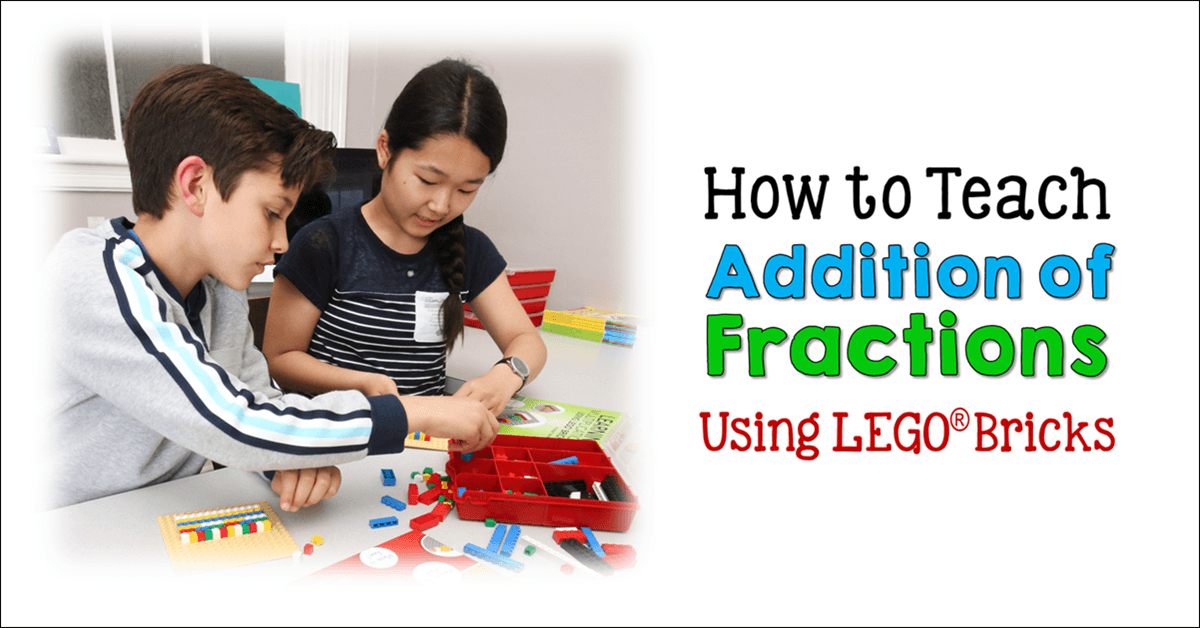# How to Teach Addition of Fractions Using LEGO BricksWe know that current math standards require students to learn through modeling using manipulatives. I have been using LEGO bricks for many years to teach students math concepts throughout the elementary and middle school curriculum. It’s a perfect math manipulative, and students love using the bricks, since many students are very familiar with them. I’ve developed specific strategies for teaching math using LEGO bricks for modeling and have been thrilled over the years to watch students’ test scores improve after they learn math using these strategies.

In recent years, I’ve taught many graduate students at High Point University how to teach with these methods, and they also report great success for their students when they use the techniques as new math teachers. I’ve recently published a series of books that show how to utilize LEGO bricks to teach all the major math topics in elementary school: Counting and Cardinality, Addition, Subtraction, Multiplication, Division, and Fractions.

Free LEGO Fractions Book
I’d like to share an example of how to teach using LEGO bricks. This is a strategy for teaching how to add fractions that have like denominators. It’s one of the lessons in my book, Teaching Fractions Using LEGO® Bricks, which is a part of my Brick Math Series. If you’d like to see more fraction lessons, you can download the entire PDF of this book as a sample of the series! Click here to request your free copy.

Teaching students to add fractions can be a challenge. Students must first understand that a fraction shows part of a whole. This method of modeling fractions with bricks helps students see clearly what the parts of the fractions mean, and how only the numerators are added, since the two fractions are part of the same whole.

Let’s add the fractions 1/6 and 2/6 together to show how the process works.

1. First, build models of the two fractions on a baseplate using LEGO bricks. The baseplate is an important component of Brick Math, because it keeps all the bricks in place.
2. Start to model the two fractions, denominators first. Use a 2×3 brick (6 studs) to model the denominator of 6. Use two 2×3 bricks that are the same color, to help students understand that the denominators are the same. Leave a little space between the two 2×3 bricks.
3. Model the numerator of the fraction 1/6 by placing a 1×1 brick above the first 2×3 brick. Model the numerator of the fraction 2/6 by placing a 1×2 brick above the second 2×3 brick. Using different color bricks for the numerators helps to show they are not the same.

1. Now it’s time to model the action of adding the two fractions. Take another 2×3 brick and place it at the bottom of a baseplate. Place the 1×1 brick above this 2×3 brick. Then place the 1×2 brick above the 1×1 brick. Your model now shows 3 studs over 6 studs. Take three 1×1 bricks and stack them on each stud of the combined numerator bricks. Have students touch each stud to count 3 as the numerator of the solution fraction of 3/6 .
1. If your students are ready for it, you can demonstrate how 3/6  = 1/2 . Place a 1×3 brick on top of the three 1×1 bricks in the model and show students that the 1×3 brick (modeling the numerator) is 1/2 the 2×3 brick (modeling the denominator).

1. The final step in the process is to have students draw their brick models on baseplate paper. Drawing the models they have built helps students reinforce the visual depiction of the mathematical concepts. Baseplate paper is included in my book, Teaching Fractions Using LEGO® Bricks, which is a free sample of my Brick Math Series books.

When you take students through the modeling process, you give them a powerful way to visualize the action of the math. For both visual and tactile learners, this method helps student understand how to add fractions that are part of the same whole.

See Two Fraction Lessons in Action on YouTube
Watch the YouTube video below to see two fraction lessons demonstrated step by step.